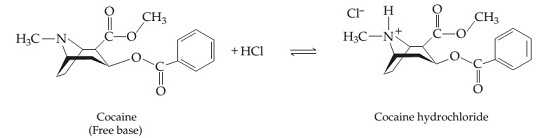# Problem: The "free-base" form of cocaine (C17H21NO4) and its protonated hydrochloride form (C17H22ClNO4) are shown below; the free-base form can be converted to the hydrochloride form with one equivalent of HCl. For clarity, not all the carbon and hydrogen atoms are shown; each vertex represents a carbon atom with the appropriate number of hydrogen atoms so that each carbon makes four bonds to other atoms.The hydrochloride form of cocaine has a solubility of 1.00 g in 0.400 mL water. Calculate the molarity of a saturated solution of the hydrochloride form of cocaine in water.

###### FREE Expert Solution

Recall that molarity is the ratio of the moles of solute and the volume of solution (in liters). In other words:

The moles of cocaine hydrochloride is:

molar mass C17H22ClNO4 = 339.806 g/mol

moles C17H22ClNO4 = 2.9428x10-3 mol

Calculate the volume of the solution: 1 mL = 10-3 L

volume = 4.0x10-4 L

We can now calculate for molarity:

95% (223 ratings)###### Problem Details

The "free-base" form of cocaine (C17H21NO4) and its protonated hydrochloride form (C17H22ClNO4) are shown below; the free-base form can be converted to the hydrochloride form with one equivalent of HCl. For clarity, not all the carbon and hydrogen atoms are shown; each vertex represents a carbon atom with the appropriate number of hydrogen atoms so that each carbon makes four bonds to other atoms.The hydrochloride form of cocaine has a solubility of 1.00 g in 0.400 mL water. Calculate the molarity of a saturated solution of the hydrochloride form of cocaine in water.